# PREVENTING DEFLECTION OF TALL STRUCTUREReading time: 1 minute

Preventing deflection of tall structure: Let us consider an example of a simple two dimensional structure. Two columns, six floors and pin joints connecting them.When we apply a wind load, we can see that the loading is shared equally between the two columns. We know that the deflection of a cantilever is given by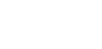So, the deflection in this case will be: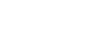Where,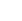is equal to the combined value of I for both columns.

If we take the same original frame, and replace the pin joints with rigid joints, the resultant structure is significantly more resistant to bending. We can see this by once again applying a wind load and seeing how it deflects as shown in figure below.The rigid joints allow the floors to act as a brace for the columns. With the pin joints most of the displacement was due to bending. By introducing the rigid floor system, this has been vastly reduced. Deflection now is mostly due to shear.

It can be shown from first principals that the deflection for such a structure is given below.

Deflection of rigid floor structure: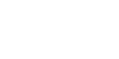One way to reduce the deflection due to shear is to include cross-members within the structure as shown in figure below. If we now apply wind load once again, we can see that the rigid floor system reduces displacement due to bending and the cross-members reduce displacement due to shear.It can be shown from first principles that the deflection for such a structure is: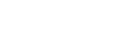That means, the deflection of this structure is 1/60000th of that of the structure with no bracing and pinned joints.